## 2.3Flow through a surface

The concept of ﬂow through a surface appears in many areas of CFD, including ﬂuid dynamics equations, numerical methods, boundary conditions and general ﬂow calculations. When we talk about something that travels through a surface, the term ﬂux is generally used.2

To quantify the ﬂux of some property, we multiply the area of surface by the property at the surface. If the property is a vector, we take the component normal to the surface. For example, the ﬂux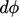associated with velocity through a surface segment of area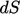would be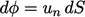.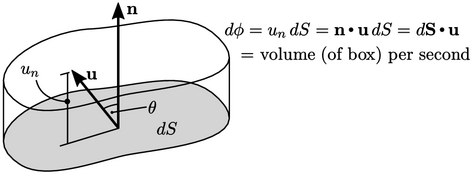As shown in the ﬁgure,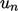can be calculated from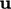by the inner product with normal vector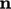of unit length, expressed as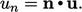(2.5)
The ﬂux associated withis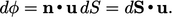(2.6)
It is a good habit to writeﬁrst since the order matters with a tensor, e.g. stress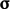, introduced in Sec. 2.6 , i.e.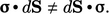### Inner product of two vectors

The normal component of velocity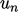is described in Eq. (2.5 ) by the inner, or “dot”, product of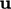and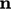. It is calculated for vectors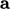and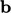as shown in the ﬁgure below, where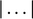denotes the magnitude of the vector and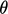is the internal angle between the two vectors.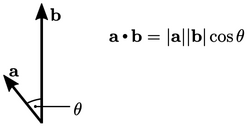The inner product of two vectors is a scalar invariant, since the magnitudes and angle are the same irrespective of the co-ordinate system used. It is calculated from vector components as follows: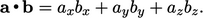The inner product of two vectors is commutative, i.e.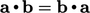. It is distributive, i.e. with an additional vector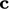,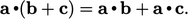Scalar multiplication and inner products are associative, i.e.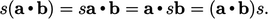An inner product of a vector with itself is simply the square of the vector magnitude, i.e.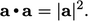(2.7)

2Joseph Fourier used the term ﬂuxion in relation to ﬂow of heat in Théorie analytique de la chaleur, 1822; James Clerk Maxwell used the term ﬂux in A Treatise on Electricity and Magnetism, 1873.

Notes on CFD: General Principles - 2.3 Flow through a surface